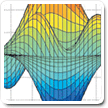Cody

# Problem 882. Solve Rubik's Cube - One Rotation

A standard Rubik's Cube is shown in 3-D and also unfolded to identify the specific Tile-face/Vector numbering.

The faces are White-Up / Red-Left / Blue-Front / Orange-Right / Yellow-Down / Green-Back (ULFRDB)

Moves are denoted as F for clockwise rotation of the Front face. F' is CCW and F2 is F twice. The loaded function r_new=rubick_rot(mov,r) implements moves 1-18: UFDLBRU'F'D'L'B'R'U2F2D2L2B2R2.```Input: (rubik)
```
```rubik: row vector of size 54
(The cube started [L=0,U=1,F=2,D=3,B=4,R=5] and then receives one face move.
```
```Output: mov (A single value that solves the cube, 1:18)
mov: is an integer 1:18 representing the moves in order: UFDLBRU'F'D'L'B'R'U2F2D2L2B2R2
```

The function rubik_rot(mov,r) is available for usage

Additional Challenges will be solving the cube at even deeper depths for time and minimizing face moves utilized.

• This easiest Rubik's Cube challenge is solely to whet everyone's appetite.
• The superflip will have to wait for a later challenge.
• Cube Theory: 20-moves Any Cube

Note: Images inserted/linked to my free google web page. Used a gif and a png.

### Solution Stats

40.43% Correct | 59.57% Incorrect
Last Solution submitted on Feb 16, 2019